# Solving the Integral of ln(x)

Lesson Transcript
Instructor
Laura Pennington

Laura received her Master's degree in Pure Mathematics from Michigan State University, and her Bachelor's degree in Mathematics from Grand Valley State University. She has 20 years of experience teaching collegiate mathematics at various institutions.

Expert Contributor
Kathryn Boddie

Kathryn has taught high school or university mathematics for over 10 years. She has a Ph.D. in Applied Mathematics from the University of Wisconsin-Milwaukee, an M.S. in Mathematics from Florida State University, and a B.S. in Mathematics from the University of Wisconsin-Madison.

Learn how the integral of ln(x) is solved. This lesson covers the steps to solve, including application and solution, and takes a look at integration by parts. Updated: 12/20/2021

## Steps to Solve

The integral of ln(x) may look simple, but it's actually a bit involved. To find this integral, we have to use integration by parts. This process is used to find the integral of a product of functions. The formula we use for integration by parts is as follows: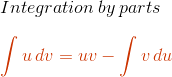Now you may look at our problem, solve the integral of ln(x), and wonder how this is a product of functions. Well, we can think of the integral of ln(x) as the integral of ln(x)*1. This way, we have a product of the functions f(x) = ln(x) and g(x) = 1.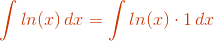Okay, now that we've cleared that up, let's look at the steps involved when using integration by parts.

1. Identify your two functions u and dv. This is usually the hardest step. One thing to help with this step is to keep in mind that we want u to be a function that is easy to find the derivative of, and we want dv to be a function for which it's easy to find the integral. Put the dx from the original integral with whichever function you named as dv.
2. Find du, or the derivative of u, and find v, or the integral of dv.
3. Plug u, v, du, and dv into the integration according to the parts formula and simplify.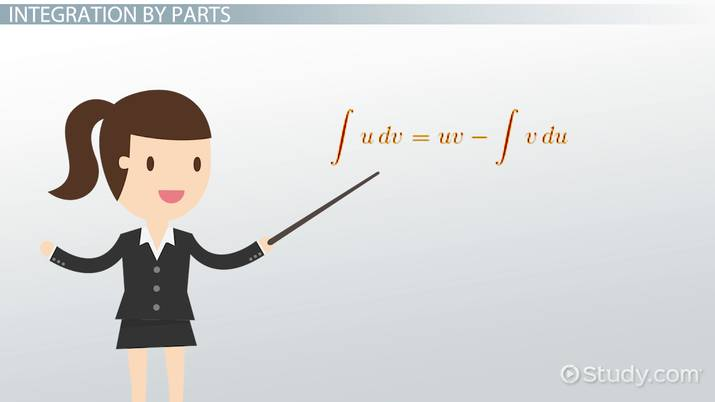An error occurred trying to load this video.

Try refreshing the page, or contact customer support.

Coming up next: Finding the Antiderivative of 1/cos(x)

### You're on a roll. Keep up the good work!

Replay
Your next lesson will play in 10 seconds
• 0:04 Steps to Solve
• 1:25 Application
• 2:21 Solution
• 2:28 Integration by Parts
Save Save

Want to watch this again later?

Timeline
Autoplay
Autoplay
Speed Speed

## Application

Let's apply these steps to the integral of ln(x). We know our two functions are ln(x) and 1. Since the derivative of ln(x) is well-known as 1/x, it would probably be a good idea to let u = ln(x). Similarly, the integral of 1 is well-known as x + C, where C is a constant. Thus, we will let dv = 1dx. It's important to note that we don't include the constants when finding different integrals during the solving process. This is because the constants that would show up throughout will all be taken care of at the end of the process when we have our final constant.

At this point, we've actually completed steps 1 and 2, and we have our u, du, v, and dv.

 u = ln(x) dv = 1dx du = (1/x)dx v = x

All we have to do now is plug the results into our formula and simplify.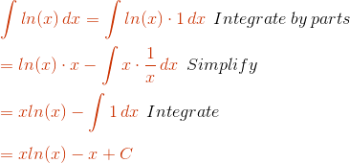## Solution

We see that the integral of ln(x) is xln(x) - x + C.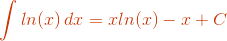## Integration by Parts

So we've found the integral of ln(x), but the use of integration by parts may be new to you, and it may have left you with some questions. To remedy this, let's take a closer look at integration by parts.

As we said, integration by parts is used to find the integral of products of functions. We can actually derive the formula for integration by parts from the product rule for derivatives. Let's see how this is done.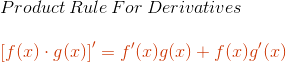Next, we'll integrate both sides of the function. You may wonder why, but this approach will all become more and more clear as we move along.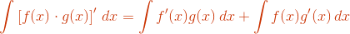To unlock this lesson you must be a Study.com Member.

## Extra Practice with the Integral of ln(x) and Integration by Parts

In the following problems, students will solve the integrals involving natural logarithms by using integration by parts. Students will also calculate a definite integral of the natural logarithm using the antiderivative of ln(x) found by integration by parts.

## Practice Problems

1. Integration by parts can be done with definite integrals as well - simply find the antiderivative first using integration by parts, and then substitute the bounds into the result. Evaluate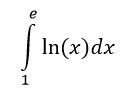2. Use integration by parts to evaluate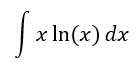3. Use integration by parts to evaluate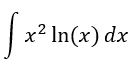4. Challenge Problem: Predict what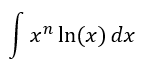will be, for non-negative n, using the results above. Then, prove that your formula is correct by using integration by parts.

## Solutions

1. We know the antiderivative of ln(x) is xln(x) - x, and so the definite integral is calculated as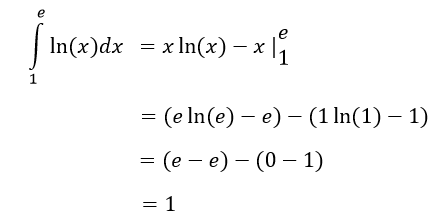2. We will use integration by parts with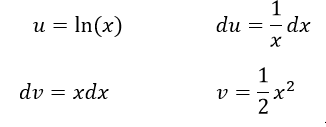Then,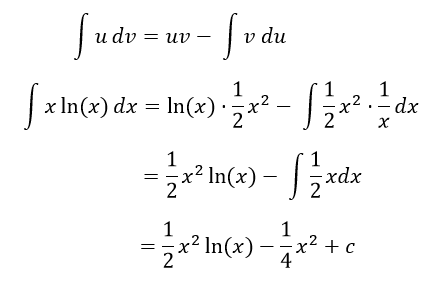3. Using integration by parts with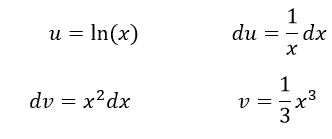we have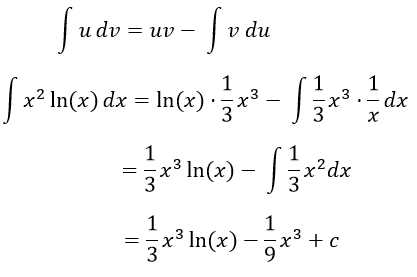4. Examining what we know already, we have that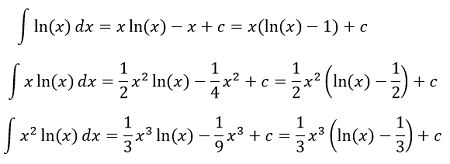So we will guess that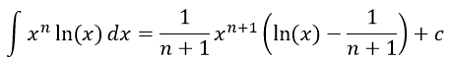To prove this, use integration by parts with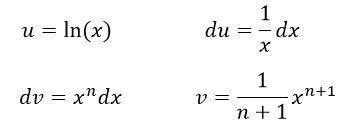to get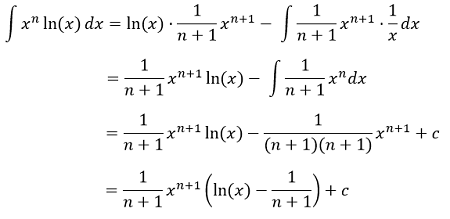### Register to view this lesson

Are you a student or a teacher?